# Arrhenius Equation Rate Constant and Temperature

## Arrhenius Equation with Example and Activation Energy Graph

Arrhenius equation is used to calculate the rate of a reaction. It is an important part of chemical kinetics. It helps in understanding the effect of temperature on the rate of a reaction. This equation was proposed in 1889 by Svante Arrhenius.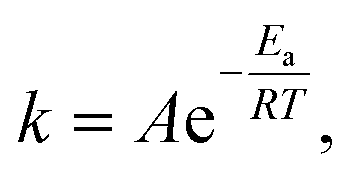Where k = Rate constant
A= Frequency factor
Ea= activation energy

R = gas constant
T = Kelvin temperature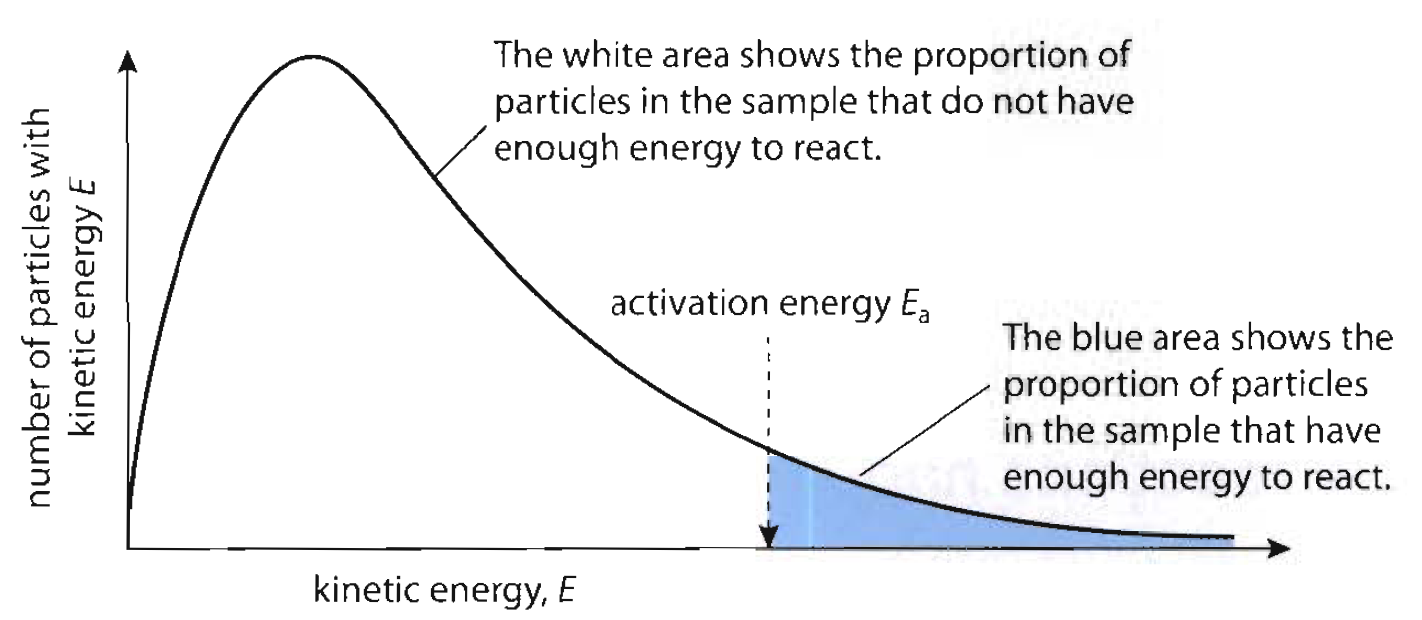The basis of the Arrhenius equation is collision theory. According to this theory, a reaction is a collision between two molecules (of the same substance or of two different substances) to form an intermediate. This intermediate so formed is unstable, which exist for a short period of time. The intermediate breaks to give two molecules of the product. The energy used in forming the intermediate is known as the activation energy.

Now, if we take to log on both sides of the Arrhenius equation, the reaction changes to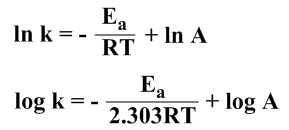Ln is natural logarithm, the values can be taken from the logarithmic table.
For graphical representation,

If we compare this equation with the equation of a straight line, we get
y = ln k
x = 1/T
m = -Ea / R
c = ln A

This gives a straight-line graph but with a negative slope.
Plotting k v/s (1/T)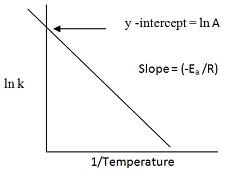EFFECT OF TEMPERATURE

From the graph, we conclude that temperature and rate if reactions are proportional. As the temperature increase, the rate of reaction also increases. Kinetic energy increases with temperature. So, when we increase the temperature the number of molecules with kinetic energy greater than the activation energy increases. This increases the rate of the overall reaction by decreases the activation energy.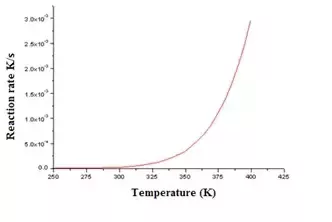For a 10 K change in temperature, the rate almost doubles.

Let’s take Arrhenius equations at time T1 and T2 where the rates of the reaction are K1 and K2 respectively.
ln k1 = -Ea/RT1+ ln A  -----(1)
ln k2 = -Ea/RT2 + ln A  -----(2)

subtracting (1) from (2)

ln k2 – ln k1 = Ea/RT1 – Ea/RT2
ln k2 / k1 = (Ea /R)[1/T1 – 1/T2]

converting ln to log;

log k2 / k1 = (Ea /2.303R)[(T2 – T1)/T1 T2]

Arrhenius equation also implies that an uncatalyzed reaction is more affected by temperature when compared to a catalyzed reaction.

SOME REAL-LIFE EXAMPLES:

• • Milk turns sour faster when kept in room temperature instead of keeping in the refrigerator;

• • Butter goes rancid faster in the summer than in the winter;

• • Eggs hard-boil much faster at sea level compared to on the mountains

• • Cold-blooded animals such as insects and reptiles tend to be more lethargic during cold days.

• EFFECT OF CATALYST

A catalyst also helps in increasing the rate of the reaction. A catalyst achieves this by reducing the activation energy required of the reaction to happen.

QUESTION

Below is a sample problem that will help you in understanding and solving questions based on the Arrhenius equation better.

• 1. Find the activation energy of the reaction (in kJ/mol) if the rate constant at 650K is 3.4 M-1 s-1 and 35.0 at 750K.

• Answer:
log k2 / k1 = (Ea /2.303R)[(T2 – T1)/T1 T2]
substituting,
log 35.0/3.4 = (Ea /2.303*8.314)[(750 – 650)/750*650]
log 10.294 = (Ea /19.147)[(100)/487500]
1.0125 = (Ea /19.147)[(100)/487500]
Ea = 1.0125*19.147*4875
Ea = 94508.395 J/mol
Ea = 9.4508 *104 J/mol
Ea = 9.4503 kJ/mol

Importance of Arrhenius equation

Arrhenius equation is important for the following reasons,

• • It allows one to account of the factors which have an impact on the rate of a reaction which cannot be determined using the rate law.

• • One can find the effect of the energy barrier, temperature, frequency, the presence of a catalyst, orientation of collisions using this equation.

• Thus these are the reasons why understanding and learning the Arrhenius equation is so important.